# Introduction to MartingalesThis is a rather important topics for anyone interested in doing Finance.
Lets look at their definition first.
A Martingale is a random processwith respect to the information filtrationand the probability distribution, iffor allfor allMartingales are used widely and one example is to model fair games, thus it has a rich history in modelling of gambling problems. If you google Martingale, you will get an image related to a Horse, because it started with Horse-betting.

We define a submartigale by replacing the above condition 2 with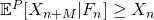for alland a supermartingale withfor all.
Take note that a martingale is both a submartingale and a supermartingale. Submartingale in layman terms, refers to the player expecting more as time progresses, and vice versa for supermartingale.

Let us try to construct a Martingale from a Random Walk now.
Letbe a random walk where the’s are IID with mean.
Let. Thenis a martingale because: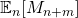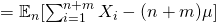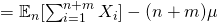since expectation distributes linearlySo how will a martingale betting strategy be like?
Here, we let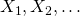be IID random variables with. We can imagineto represent the result of a coin-flipping game where,
– player win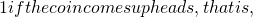latex X_i = 11 if the coin comes up tails, that is,Consider further now a doubling strategy where we keep doubling the bet until we eventually win. Once we win, we stop and our initial bet islatex n^{th}latex 2^{n-1}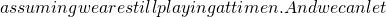latex W_n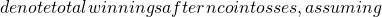latex W_0 = 0latex W_nlatex W_n \in \{ 1, -2^n +1 \}latex n^{th}latex W_n = -(1 + 2 + \ldots + 2^{n-2}) + 2^{n-1}latex = -(2^{n-1} – 1) + 2^{n-1} = 1latex W_n = -(1 + 2 + \ldots + 2^{n-1}) = -2^n +1latex W_nlatex \mathbb{E}[W_{n+1} | W_n] = W_nlatex W_n = 1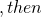latex P(W_{n+1} = 1 | W_n = 1) = 1latex \mathbb{E}[W_{n+1} | W_n = 1] = 1 = W_nlatex W_n = -2^n +1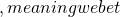latex 2^n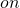latex (n+1)^{th}latex W_{n+1} \in \{ 1 , -2^{n+1} + 1 \}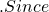latex P(W_{n+1} = 1) | W_n = -2^n +1) = \frac{1}{2}latex P(W_{n+1} = -2^{n+1}+1 | W_n = -2^n +1) = \frac{1}{2}latex \mathbb{E}[W_{n+1} | W_n = -2^n +1] = \frac{1}{2} \times 1 + \frac{1}{2} \times (-2^{n+1} +1) = -2^n + 1 = W_nlatex \mathbb{E} [W_{n+1} | W_n] = W_nlatex X_nlatex P(X_{n+1} = k+1 | X_n = k) = \frac{k}{n + 2}latex P(X_{n+1} = k | X_n = k) = \frac{n + 2 – k}{n + 2}latex M_n := \frac{X_n}{n+2}\$ is a martingale.

Not readable? Change text.